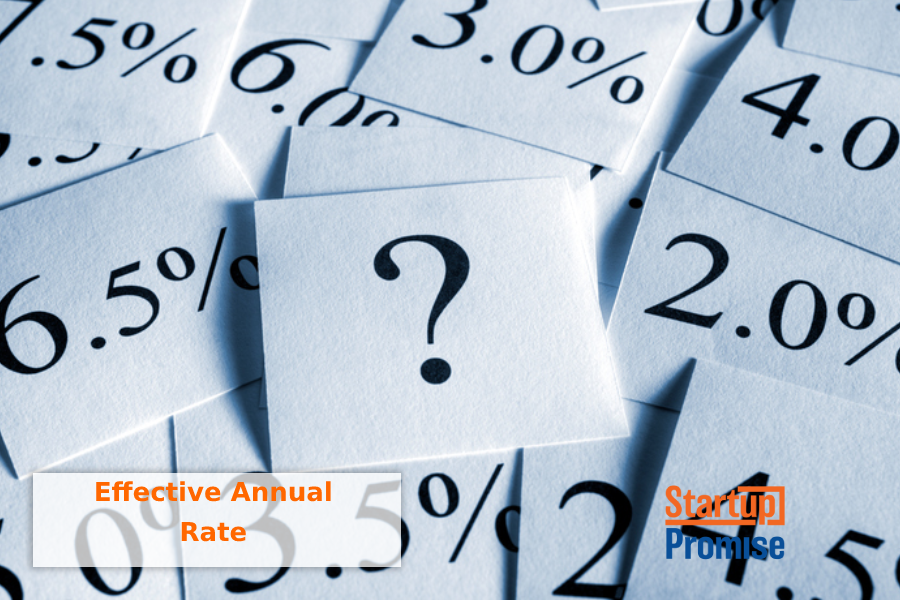# Effective Annual RateLatest posts by Holly Magister, CPA, CFP® (see all)

The effective annual rate – also called the effective interest rate, the effective rate, or the annual equivalent rate – describes the amount of interest paid or earned on an investment or loan as the result of compounding over the period of a year or some annual timeframe.

Typically, the interest given for an investment or loan is called the nominal interest rate. However, when interest is compounded more than once per year, the amount of interest actually earned in the case of an investment, or expensed in the case of a loan, will be higher than the nominal interest rate.  This higher rate is called the effective annual rate.

## Why the Effective Annual Rate is Necessary

The effective annual rate is used to compare financial products or investments that compound interest differently over time. As an example, the effective annual rate can be used to compare an investment that pays 12% compounded monthly with one that pays 12.2% compounded semi-annually. Using the effective annual rate, a business owner, investor, bank, other lending institution or individual can determine which investment will earn or cost more on an annual basis. The effective annual rate allows an apples-to-apples comparison.

## Calculating the Effective Annual Rate

The effective annual rate is calculated by taking the nominal interest rate (the rate denoted on the loan, investment, or other financial product), and adjusting it for the total number of compounding periods that it will experience in its lifetime.

Effective Annual Rate = (1 + (nominal rate/number of compounding periods)) ^ (number of compounding periods) – 1

In the example above, we might conclude that the investment with the higher nominal interest rate of 12.2% would yield a higher return annually, however the formula would show us that because it is compounded fewer times over the time period (only twice instead of monthly), the investment with a nominal interest rate of 12% would actually yield the higher return.

## Effective Annual Rate and Compounding

We can assume that as as the number of compounding periods increases, so does the effective annual rate (assuming the interest rate is the same). An investment that compounds monthly would yield a higher return than one that compounds quarterly and one that compounds quarterly will yield a higher return than one that compounds semi-annually.

The formula allows us to compare different stated or nominal rates with different compounding periods to determine the investment that will offer the best return. Similarly, computing the Effective Annual Rate of interest when obtaining a loan allows the borrower to compare different bank loans to determine which is the least costly.

Click to rate this post!
[Total: 1 Average: 5]